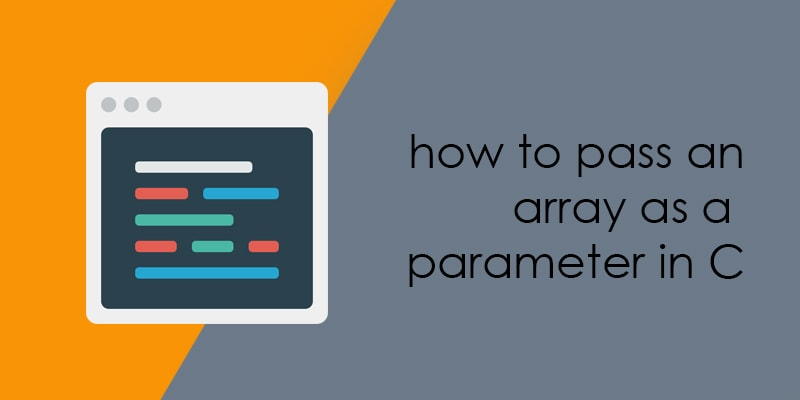# How to pass an array as a parameter in C?An array is a collection of similar type of data, data could be value or address. When we pass an array as a parameter then it splits into the pointer to its first element. We can say that if I shall pass the array of character as a parameter then it would be split into the pointer to the character. So, if a function parameter declared as T arr[] or T arr[n] is treated as T *arr.

In C language, it is easy to work on the 1D array as compared to a multidimensional array. In this article, I will explain few ways to pass the array as parameters.

#### There are a lot of ways to pass the 1D array as arguments

Let’s see an example code to how to pass a 1D array as a parameter.In the example program, I am creating an integer array and passing this integer array to the function as the parameter for the tracing the array elements. The function parameter type could be as int aiData[] or int aiData[n] is treated as int *aiData.

##### Parameters as a pointer

We have already know that array split into the pointer of its first element, so here I am creating a function whose parameters is an integer pointer.##### Parameters as a sized array

One of the simple ways to pass the array as a parameter declared the function with prototype same as the array that will pass to the function.

##### Parameters as an unsized array

When we pass the 1D array as a parameter then don’t need to specify the size of the array. It behaves like T *, where T is the type of the array.

#### Ways to passing a 2D array as parameter to the function

Similar to the 1D array we can pass the 2D array as a parameter to the function. It is important to remember that when we pass a 2D array as a parameter decays into a pointer to an array, not a pointer to a pointer.

##### Passing 2d array to function in c using pointers

The first element of the multi-dimensional array is another array, so here, when we will pass a 2D array then it would be split into a pointer to the array.

For example,
If int aiData, is a 2D array of integers, it would be split into a pointer to the array of 3 integers (int (*)).

##### Passing 2d array to function with row and column

In which prototype of the function should be same as the passing array. In another word, we can say that if int aiData is a 2D array, the function prototype should be similar to the 2D array.

##### Passing 2d array to function omitting the row

In C language, the elements of the 2d array are stored row by row, there is no much more importance of the row when we are passing a 2d array to function. But it needs to remember that we have to specify the size of the column because it is used in jumping from row to row in memory.

##### Passing 2d array to function, using the pointer to 2D array

If int aiData is a 2D array of integers, then &aiData would be pointer the 2d array that has 3 row and 3 column.## One comment

1.Tim says:

if you do not know how many rows are in a 2D array how can you determine how many rows are in an 2D array?